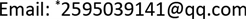﻿ 一类拟线性椭圆方程零点的临界群 Critical Groups of Zeros for a Quasilinear Elliptic Equation

Advances in Applied Mathematics
Vol.07 No.07(2018), Article ID:25984,6 pages
10.12677/AAM.2018.77101

Critical Groups of Zeros for a Quasilinear Elliptic Equation

Zhenting Wang*, Mingshuang Duan, Weiwei Li, Yaqiong Wang

College of Sciences, North China University of Technology, BeijingReceived: Jun. 23rd, 2018; accepted: Jul. 13th, 2018; published: Jul. 20th, 2018ABSTRACT

In this paper, we will study a class of quasilinear elliptic equations by Morse theory. Compared with the existing results, our new contents include two aspects: we first get the isolation of the zero when the nonlinear is resonant. Secondly, the critical groups for the zero are obtained under the weak condition.

Keywords:Quasilinear Elliptic Equations, Resonance, Morse TheoryCopyright © 2018 by authors and Hans Publishers Inc.

This work is licensed under the Creative Commons Attribution International License (CC BY).

http://creativecommons.org/licenses/by/4.0/1. 引言

2. 已有结果与本文定理

$\left\{\begin{array}{l}-{\Delta }_{p}u-\Delta u=f\left(x,u\right),x\in \Omega \\ u=0,x\in \partial \Omega ,\end{array}$ (P)

(f1) $f\left(x,0\right)=0,f\in C\left(\overline{\Omega }×R,R\right)$ 并且

$|f\left(x,u\right)|\le c\left(1+{|u|}^{\mu -1}\right),\text{ }\text{ }\text{ }\text{ }\text{ }\text{ }\text{ }\text{ }\text{ }\forall u\in R,x\in \Omega ,$

$I\left(u\right)=\frac{1}{p}{\int }_{\Omega }{|\nabla u|}^{p}\text{d}x+\frac{1}{2}{\int }_{\Omega }{|\nabla u|}^{2}\text{d}x-{\int }_{\Omega }F\left(x,u\right)\text{d}x$

$‖u‖={‖\nabla u‖}_{p}={\left({\int }_{\Omega }{|\nabla u|}^{p}\text{d}x\right)}^{\frac{1}{p}}.$

$K=\left\{u\in E|{J}^{\prime }\left(u\right)=0\right\},{J}^{c}=\left\{u\in E|J\left(u\right)\le c\right\},{K}_{c}=\left\{u\in K|J\left(u\right)=c\right\}$

${C}_{q}\left(J,{u}_{0}\right):={H}_{q}\left({J}^{c}\cap U,{J}^{c}\cap U\\left\{{u}_{0}\right\}\right),q\in N$

$0<{\lambda }_{1}<{\lambda }_{2}<\cdots <{\lambda }_{k}<\cdots$

(g1) $f\in {C}^{1}\left(\overline{\Omega }×R,R\right)$ ，满足

${\lambda }_{k}<{f}^{\prime }\left(x,0\right)<{\lambda }_{k+1}$

${C}_{q}\left(I,0\right)={\delta }_{q,k}G$

(g2) $f\in {C}^{1}\left(\overline{\Omega }×R,R\right)$ ，满足 ${f}^{\prime }\left(x,0\right)={\lambda }_{1}$ 及存在常数 $\alpha >0$ 使得

$F\left(x,u\right)\le \frac{{\lambda }_{1}}{2}{u}^{2},\text{ }\text{ }\text{ }\text{ }\text{ }|u|\le \alpha$

${C}_{q}\left(I,0\right)={\delta }_{q,0}G$

$g\left(x,u\right)=f\left(x,u\right)-{\lambda }_{1}u$

(f2) $\underset{|u|\to 0}{\mathrm{lim}}\frac{g\left(x,u\right)}{u}=0$ ，并且存在常数 $\alpha >0$$C>0$$2 使得

$g\left(x,u\right)u\le C{|u|}^{\gamma },\text{ }\text{ }\text{ }\text{ }\text{ }|u|\le \alpha$

${C}_{q}\left(I,0\right)={\delta }_{q,0}G$

2) 在之前的文章中，为了使用临界群，一般都是假设零点是孤立的临界点，而在我们的结果中可以证明此结论；

3) 根据条件(f2)，可以推出存在常数 $C>0$ 使得

$F\left(x,u\right)-\frac{{\lambda }_{1}}{2}{u}^{2}\le C{|u|}^{\gamma },\text{ }\text{ }\text{ }\text{ }|u|\le \alpha$

3. 定理的证明

${‖{u}_{n}‖}_{C\left(\Omega \right)}=\underset{n}{\mathrm{max}}|{u}_{n}|\to 0$

$g\left(x,{u}_{n}\right){u}_{n}\le C{|{u}_{n}|}^{\gamma },\text{ }\text{ }\text{ }\text{ }\text{ }\text{ }|{u}_{n}|\le \alpha$

$\begin{array}{c}{\lambda }_{1}{\int }_{\Omega }{|{u}_{n}|}^{2}\text{d}x\le {\int }_{\Omega }{|\nabla {u}_{n}|}^{2}\text{d}x\\ =-{\int }_{\Omega }{|\nabla {u}_{n}|}^{p}\text{d}x+{\lambda }_{1}{\int }_{\Omega }{|{u}_{n}|}^{2}\text{d}x+{\int }_{\Omega }g\left(x,{u}_{n}\right){u}_{n}\text{d}x\\ \le -{‖{u}_{n}‖}^{p}+{\lambda }_{1}{\int }_{\Omega }{|{u}_{n}|}^{2}\text{d}x+C{\int }_{\Omega }{|{u}_{n}|}^{\gamma }\text{d}x\\ \le -{‖{u}_{n}‖}^{p}+{\lambda }_{1}{\int }_{\Omega }{|{u}_{n}|}^{2}\text{d}x+C{‖{u}_{n}‖}^{r}\end{array}$

${\lambda }_{1}{\int }_{\Omega }{|{u}_{n}|}^{2}\text{d}x\le {\int }_{\Omega }{|\nabla {u}_{n}|}^{2}\text{d}x<{\lambda }_{1}{\int }_{\Omega }{|{u}_{n}|}^{2}\text{d}x$

1) ${\Phi }_{\tau }$ 在邻域U中满足(PS)条件；

2) 对于 $\forall \tau \in \left[0,1\right]$${u}_{0}$${\Phi }_{\tau }$ 在邻域U中的唯一临界点；

3) 算子 $\tau \to {\Phi }_{\tau }$${C}^{1}\left(U\right)$ 光滑的；

${C}_{q}\left({\Phi }_{0},{u}_{0}\right)={C}_{q}\left({\Phi }_{1},{u}_{0}\right),\text{ }\text{ }\text{ }\text{ }\text{ }\text{ }\forall q\in N$

$\theta \left(u\right)=\left\{\begin{array}{l}u,\text{ }\text{ }\text{ }\text{ }\text{ }\text{ }\text{ }\text{ }\text{ }\text{ }\text{ }\text{ }\text{ }\text{ }\text{ }\text{ }\text{ }\text{ }\text{ }|u|\le \alpha /2,\\ \alpha ,\text{ }\text{ }\text{ }\text{ }\text{ }\text{ }\text{ }\text{ }\text{ }\text{ }\text{ }\text{ }\text{ }\text{ }\text{ }\text{ }\text{ }\text{ }\text{ }u\ge \alpha ,\\ -\alpha ,\text{ }\text{ }\text{ }\text{ }\text{ }\text{ }\text{ }\text{ }\text{ }\text{ }\text{ }\text{ }-u\ge \alpha ,\end{array}$

${\Phi }_{\tau }\left(u\right)=\frac{1}{2}{\int }_{\Omega }{|\nabla u|}^{2}\text{d}x+\frac{1}{p}{\int }_{\Omega }{|\nabla u|}^{p}\text{d}x-\frac{{\lambda }_{1}}{2}{\int }_{\Omega }{|u|}^{2}\text{d}x-{\int }_{\Omega }G\left(x,\left(1-\tau \right)u+\tau \theta \left(u\right)\right)\text{d}x$

${‖{u}_{n}‖}_{C\left(\Omega \right)}=\underset{n}{\mathrm{max}}|{u}_{n}|\to 0$

$\theta \left({u}_{n}\right)={u}_{n}$

$0={{\Phi }^{\prime }}_{{\tau }_{n}}\left({u}_{n}\right)={{\Phi }^{\prime }}_{0}\left({u}_{n}\right)={I}^{\prime }\left({u}_{n}\right)$

${u}_{n}$ 为泛函I的非零临界点，这与引理1.2矛盾。证毕。

${C}_{q}\left({\Phi }_{1},0\right)={\delta }_{q,0}G$

$|\theta \left(u\right)|\le \alpha ,\text{ }\text{ }\text{ }\text{ }\text{ }\text{ }\text{ }\forall u\in {W}_{0}^{1,p}\left(\Omega \right)$

$\begin{array}{c}{\Phi }_{1}\left(u\right)=\frac{1}{2}{\int }_{\Omega }{|\nabla u|}^{2}\text{d}x+\frac{1}{p}{\int }_{\Omega }{|\nabla u|}^{p}\text{d}x-\frac{{\lambda }_{1}}{2}{\int }_{\Omega }{|u|}^{2}\text{d}x-{\int }_{\Omega }G\left(x,\theta \left(u\right)\right)\text{d}x\\ \ge \frac{1}{p}{\int }_{\Omega }{|\nabla u|}^{p}\text{d}x-C{\int }_{\Omega }{|u|}^{\gamma }\text{d}x\\ \ge C{‖u‖}^{p}-C{‖u‖}^{\gamma }\end{array}$

${C}_{q}\left(I,0\right)={C}_{q}\left({\Phi }_{0},0\right)={C}_{q}\left({\Phi }_{1},0\right)={\delta }_{q,0}G$

4. 结语

Critical Groups of Zeros for a Quasilinear Elliptic Equation[J]. 应用数学进展, 2018, 07(07): 842-847. https://doi.org/10.12677/AAM.2018.77101

1. 1. Benci, V., Fortunato, D. and Pisani, L. (1998) Soliton-Like Solutions of a Lorentz Invariant Equation in Dimension 3. Journal of Mathematical Physics, 3, 315-344. https://doi.org/10.1142/S0129055X98000100

2. 2. Dinca, G., Jebelean, P. and Mawhin, J. (2001) Variational and Topological Methods for Dirichlet Problems with p Laplacian. Portugal. Math. (N.S), 58, 339-378.

3. 3. Chang, K. (1993) Infinite Dimensional Morse Theory and Multiple Solution Problems. Birkhauser. https://doi.org/10.1007/978-1-4612-0385-8

4. 4. 张恭庆. 临界点理论及其应用[M]. 上海: 上海科学技术出版社, 1986.

5. 5. Cingolani, S. and Vannella, G. (2003) Critical Groups Computations on a Class of Sobolev Banach Spaces via Morse Index. Annales de l’Institut Henri Poincare. Analyse Non Lineaire, 20, 271-292. https://doi.org/10.1016/S0294-1449(02)00011-2

6. 6. Sun, M.Z., Zhang, M.L. and Su, J.B. (2015) Critical Groups at Zero and Multiple Solutions for a Quasilinear Elliptic Equation. Journal of Mathematical Analysis and Applications, 428, 696-712. https://doi.org/10.1016/j.jmaa.2015.03.033

7. 7. Sun, M. (2012) Multiplicity of Solutions for a Class of the Quasilinear Elliptic Equations at Resonance. Journal of Mathematical Analysis and Applications, 386, 661-668. https://doi.org/10.1016/j.jmaa.2011.08.030

8. 8. Chang, K.-C. and Ghoussoub, N. (1996) The Conley Index and the Critical Groups via an Extension of Gromoll-Meyer Theory. Topol. Methods Nonlinear Analysis, 7, 77-93. https://doi.org/10.12775/TMNA.1996.003

9. 9. Iannizzotto, A., Liu, S., Perera, K. and Squassina, M. (2016) Existence Results for Fractional p-Laplacian Problems via Morse Theory. Advances in Calculus of Variations, 9, 101-125. https://doi.org/10.1515/acv-2014-0024